# Logical Venn Diagram

Venn diagrams are used to visualise logical relationship among given groups of elements.

In this chapter, we deal with the questions which aim at analysing a candidate's ability to relate a given groups of items and illustrate it diagrammatically. Different geometrical figures like circle, triangles, squares and rectangles, etc. are used to represent these diagrams.

## Types of Questions

Based on the variety of questions that are asked in various competitive exams, we have classified logical venn diagrams into two types as follows

Type #1:
Identification of Venn Diagram

In this type of questions, we deal with different types of cases which are as follows

Case (I) If two separate groups of items are completely unrelated to each other but they are all completely included in the third group, then the relationships can be diagrammatically shown as in the given example.

Example 1: Vehicle, Car Bus

Solution:  Venn diagram would be as follows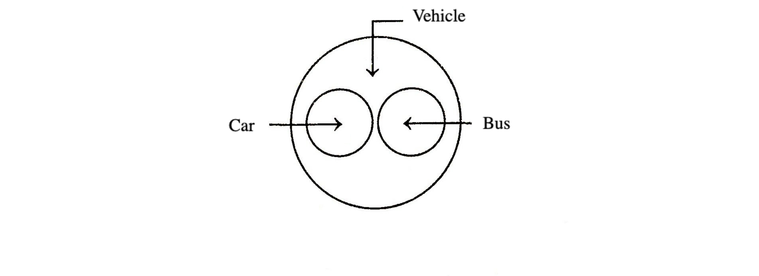Bus and car are entirely different but both are vehicles.

Example 2: Hospital, Nurse, Patient

Solution:  Venn diagram would be as follows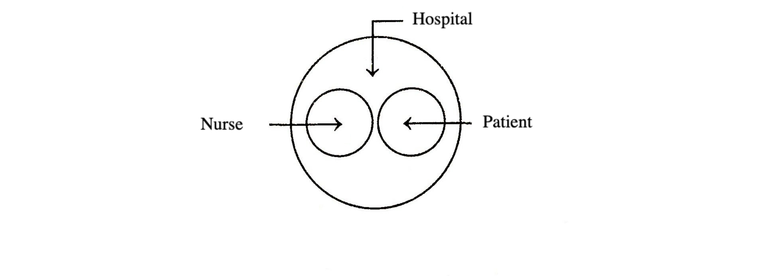Nurse and patient are entirely different but both are present in hospital.

Case (II) When two groups of items have some common relationship and both of them are completely included in the third group, the relationships are shown by two smaller intersecting circle in a third large circle.

Example 3: Lawyers, Teacher, Educated

Solution:  Venn diagram would be as follows

Some lawyers are teachers and some teachers are lawyers but all lawyers and teachers are educated.Case (III) If one item belongs completely to the class of second while, third item is entirely different from the two, then they may be represented by the given below diagram in the example.

Example 4: Engineers, Human Beings, Rats

Solution:  Venn diagram would be as follows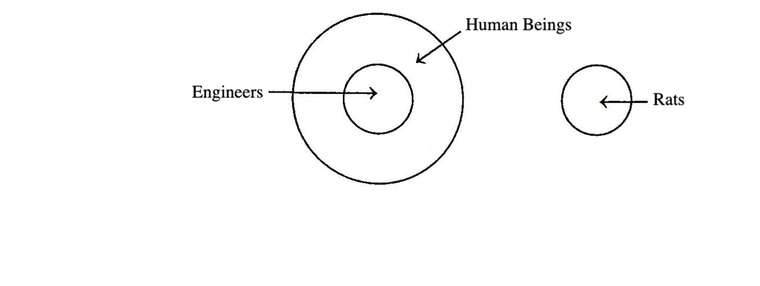We know that, all engineers are human beings but rats are entirely different from both of these.

Case (IV) If one group of items is partly included in the second group of items and the third group is completely unrelated to these two groups, then their relationship is diagrammatically show as in the following example.

Example 5: Wire, Copper, Paper

Solution:  Venn diagram would be as follows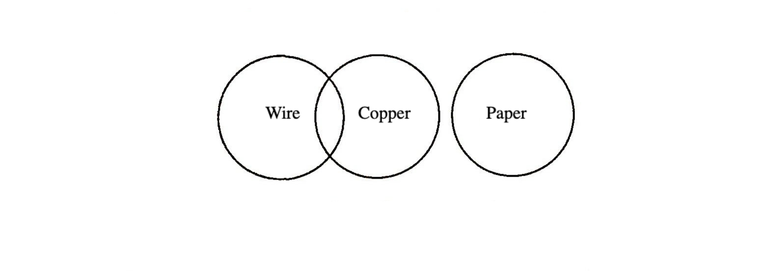Show wires are made of copper but paper is entirely different.

Case (V) If one item belongs to the class of second and the third item is partly related to these two, then their relationships is as shown in the following example..

Example 6: Female, Mother, Doctors

Solution:  Venn diagram would be as followsAll mothers are females but some females and some mothers can be doctors. So, the circle representing doctors would intersect both of the two concentric circles.

Example 7: Doctor, Educated, Employed

Solution:  Venn diagram would be as followsAll the doctors are educated but some are employed.

Case (VI) If one item belongs to the class of second and the third item is partly related to the second, then their relationship is as shown in the following example.

Example 8: Male, Fathers, Children

Solution:  Venn diagram would be as follows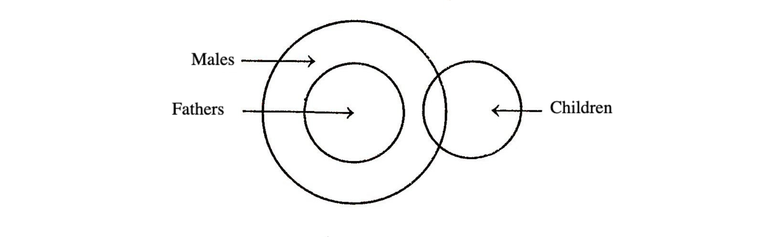All fathers are males. This would be represented by two concentric circles but some males are children and children cannot be fathers.

Case (VII) If the items evidently belong to three different groups, then they are represented by three non-intersecting circles.

Example 9: Doctors, Lawyers, Engineers.

Solution:  Venn diagram would be as follows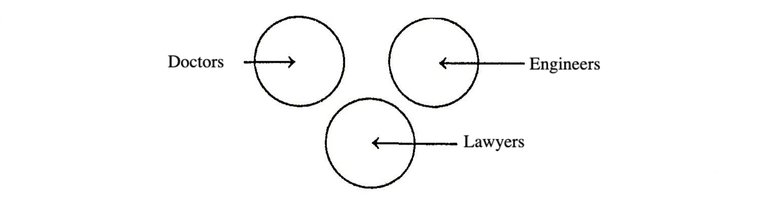These three have no relationship between each other.

So, they are represented by three disjoint circles.

Case (VIII) If one item belongs to the class of second and the second belongs to the class of third, then they are represented by three concentric circles.

Example 10: Second, Minutes, Hours

Solution:  Venn diagram would be as follows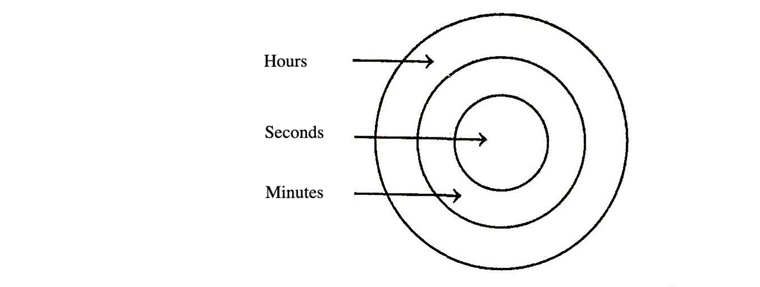Clearly, hour consists of minutes and minutes consists of seconds.

Case (IX) If two items are partly related to the third and are themselves independent of each other, then they are represented by three intersecting circle in a line.

Example 11: Dogs, Pets, Cats

Solution:  Venn diagram would be as followsClearly, some dogs and some cats are pets. But all the pets are not dogs or cats. Also dogs and cats are not related to each other.

Case (X) If the three are partly related to each other, then they are represented as shown in the following example.

Example 12: Married, Women, Teachers

Solution:  Venn diagram would be as follows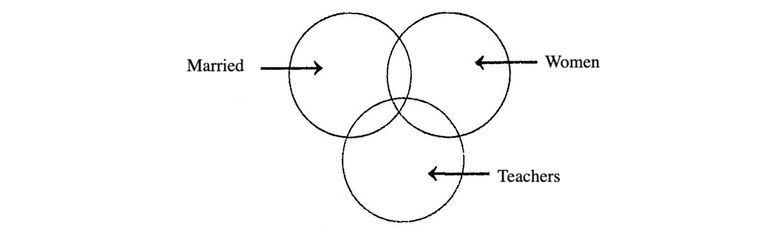Some women are teachers. Some women are married. Some teachers are married. Some married women are teachers..

Type #2:
Analysis of Venn Diagram

In this type of questions, generally a Venn diagram comprising of different geometrical figures is given. Each geometrical figure in the diagram represents a certain class. The candidate is required to study and analyse the figure carefully and then answer the given questions based on it.

Directions (Example Nos. 13-14) Study the following diagram to answer these questions.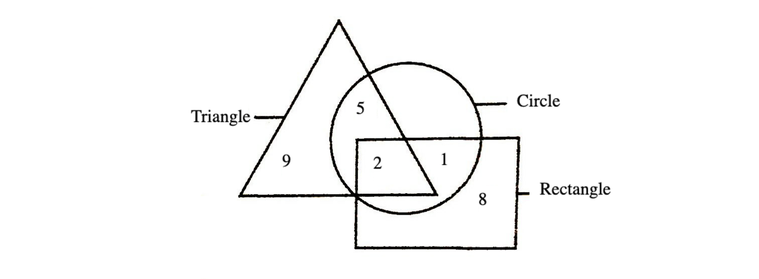Example 13: Find out the number, that lies inside all the figures.

(a) 2                              (b) 5
(c) 9                              (d) No such number is there

Solution:  (a) Such number should belong to all three figures, i.e. circle, rectangle and triangle. There is only one number, i.e. 2 which belongs to all three figures.

Example 14: What are the number that lie inside any two figures?

(a) 2, 1                              (b) 5, 1
(c) 5, 9                              (d) 9, 1

Solution:  (b) Such numbers are 5 and 1 because 5 lies inside the triangle and circle. And, 1 lies inside the circle and rectangle.

Directions (Example Nos. 15-16) Study the figure given below carefully and answer the questions that follow.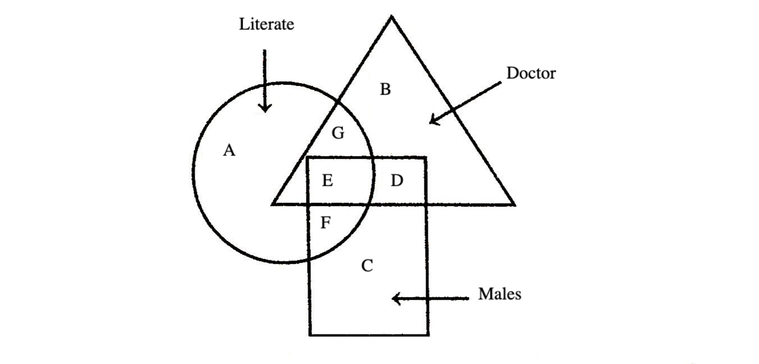Example 15: Which part shows Literate Males, who are Doctors?

(a) G                             (b) E
(c) D                             (d) F

Solution:  (b) To depict the required part, the figure should show the region common to all the three diagrams, i.e. E. E shows literate Males, who are Doctors.

Example 16: Which part shows Males, who are neither Doctor nor Literate?

(a) A          (b) B          (c) C         (d) D

Solution:  (c) To depict the required part, the figure should show the region common to only rectangle, i.e. C. C shows Males, who are neither Doctor nor Literate.

Example 17: Which one of the areas marked I-VII represents the urban educated who are not hard working?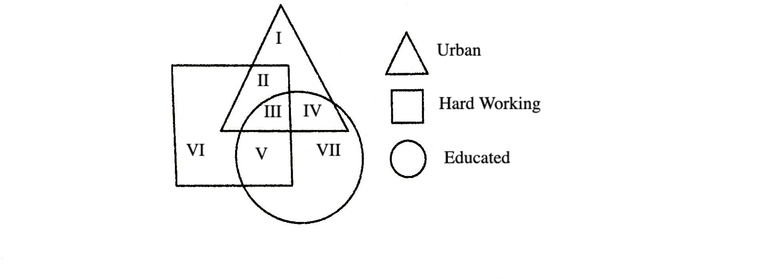(a) II          (b) I         (c) IV           (d) V

Solution:  (c) The area which represents the urban educated who are not hard working lies outside the square but common to triangle and circle, i.e. IV.

LearnFrenzy provides you lots of fully solved "Logical Venn Diagrams" Questions and Answers with explanation.